# MCAT Physical : Factors Affecting Reaction Rate

## Example Questions

### Example Question #1 : Factors Affecting Reaction Rate

For any given chemical reaction, one can draw an energy diagram. Energy diagrams depict the energy levels of the different steps in a reaction, while also indicating the net change in energy and giving clues to relative reaction rate.

Below, a reaction diagram is shown for a reaction that a scientist is studying in a lab. A student began the reaction the evening before, but the scientist is unsure as to the type of the reaction. He cannot find the student’s notes, except for the reaction diagram below.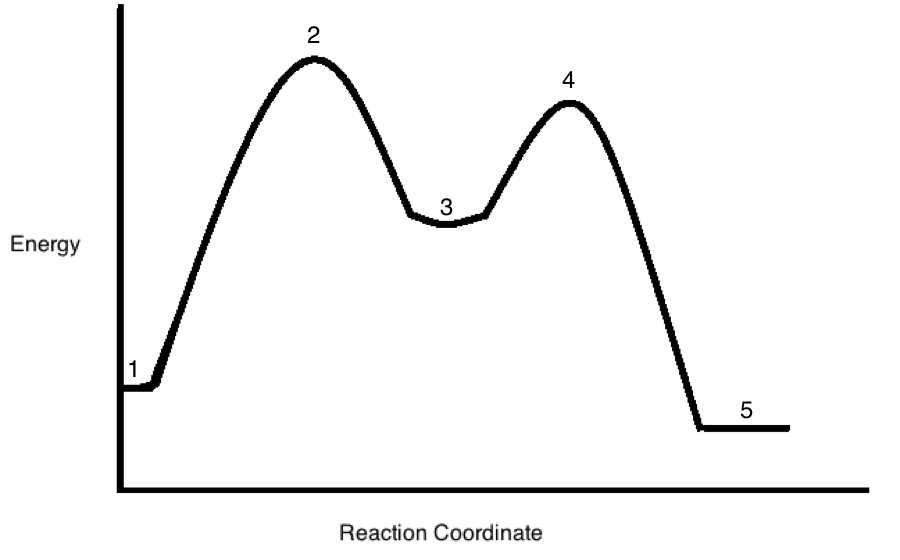What factors, not depicted in the reaction diagram, are most likely to influence reaction rate?

Reactant stability

Product stability

Reactant concentrations

Intermediate stability

Free energy change

Reactant concentrations

Explanation:

Reactant concentration, along with activation energy and temperature, is a major determinant in reaction rate, as demonstrated in Le Chatlier's principle.

### Example Question #2 : Factors Affecting Reaction Rate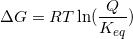Given the above equation for a reaction, which statement must ALWAYS be true?

If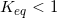, the reaction is spontaneous

If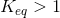, the reaction is spontaneous

If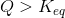, the reaction is spontaneous

If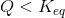, the reaction is spontaneous

If, the reaction is spontaneous

Explanation:

This question can be approached two different ways. One way is by looking at the equation, and the other is understanding what the variables in the equation represent.

1) The first method is understanding the equation. Because we must understand basic log and natural log functions, we know that the natural log of a fraction is a negative number. Additionally, because we know that a reaction is spontaneous when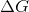is negative, we can conclude that a reaction will be spontaneous when Q is less than Keq. R is a positive constant, and T cannot be negative.

2) A second approach to this question is understanding the variables in the equation. Keq is the ratio of product to reactant at equilibrium. Q is the reaction quotient, and represents the ratio of product to reactant at a given point in time. If Q is less than Keq, the reaction is still too far to the left, and is still reacting to reach equilibrium. Because the reaction is trying to reach equilibrium, it will continue to spontaneously react until it reaches that point.

### Example Question #3 : Factors Affecting Reaction Rate

Consider the energy diagram of a three-step chemical process shown below. Which step would have the fastest rate?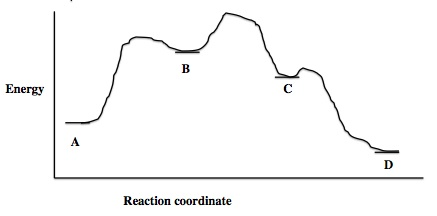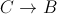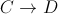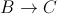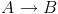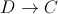Explanation:

The reaction with the fastest rate will have the lowest activation energy; the energy difference between the starting compound and the top of the energy barrier that must be overcome to proceed to the product should be the smallest. Of the given choices, the reaction proceeding from C to D has the lowest energy barrier, and hence will have the lowest activation energy and fastest reaction rate.

In contrast, the energy required to move from point A to point B has the largest energy barrier, and can be assumed to be the rate-limiting step.

### Example Question #4 : Factors Affecting Reaction Rate

A scientist is studying a reaction, and places the reactants in a beaker at room temperature. The reaction progresses, and she analyzes the products via NMR. Based on the NMR readout, she determines the reaction proceeds as follows: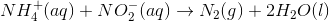In an attempt to better understand the reaction process, she varies the concentrations of the reactants and studies how the rate of the reaction changes. The table below shows the reaction concentrations as she makes modifications in three experimental trials.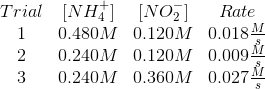Reaction rates depend on reaction concentrations, as well as the reaction constant. The Arrhenius equation is used to calculate this constant. Which of the following factors is used in the Arrhenius equation?

I. Temperature

II. Activation energy

III. Reactant concentrations

I and II

I only

II and III

I, II, and III

III only

I and II

Explanation:

If you thought all of these factors are used in the Arrhenius equation, consider that the rate constant is independent of the reactant concentrations. The concentration effect is considered in the rate law by multiplying the concentrations by the constant, but the concentrations themselves are independent of the actual Arrhenius calculation.

The calculation shows that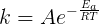. In this equation,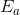is the activation energy and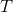is the temperature.

### Example Question #5 : Factors Affecting Reaction Rate

A scientist is studying a reaction, and places the reactants in a beaker at room temperature. The reaction progresses, and she analyzes the products via NMR. Based on the NMR readout, she determines the reaction proceeds as follows:In an attempt to better understand the reaction process, she varies the concentrations of the reactants and studies how the rate of the reaction changes. The table below shows the reaction concentrations as she makes modifications in three experimental trials.The scientist in the passage calculates that, under experimental conditions, the gievn reaction releases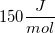before it comes to equilibrium.

Under the exact same conditions, another unknown reaction is studied. This second reaction releases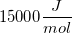before it comes to equilibrium.

Which of the following must be true?

The first reaction will always progress faster

The second reaction will always progress faster

We cannot predict the relative rates of these two reactions with the information given

The first reaction will progress faster at the experimental conditions, but may be slower at other conditions

The second reaction will progress faster at the experimental conditions, but may be slower at other conditions

We cannot predict the relative rates of these two reactions with the information given

Explanation:

The amount of energy released or absorbed by a reaction is independent of its rate. Rate is principally determined by the activation energy, while the inherent bond energies of reactants and products dictate thermodynamic changes. One cannot be used to predict the other.

### Example Question #6 : Factors Affecting Reaction Rate

In any given reaction, the rate-determining step __________.

cannot be modified by use of a catalyst

may change if temperature is changed or pressure is changed

represents the step with the lowest activation energy

represents the endothermic component of the reaction

may change if temperature is changed or pressure is changed

Explanation:

Reaction rates, including the rate-determining step, can be altered with a change in conditions. This includes a change in temperature, a change in pressure, or the addition of a catalyst.

### Example Question #7 : Factors Affecting Reaction Rate

For a multi-step chemical reaction, which of the following will reduce the reaction rate?

A step with a high energy transition state

A step that involves the limiting reagent

A step with a high activation energy

A step that involves the excess reagent

A step with a high activation energy

Explanation:

For a multi-step reaction, the rate-determining step is the step with the highest activation energy. Be careful not to confuse this with the energy transition state.

### Example Question #8 : Factors Affecting Reaction Rate

What is the role of a catalyst in an reaction?

To decrease the rate of reaction by lowering the activation energy

To increase the rate of reaction by increasing the activation energy

To increase the rate of reaction by lowering the activation energy

To decrease the rate of reaction by increasing the activation energy